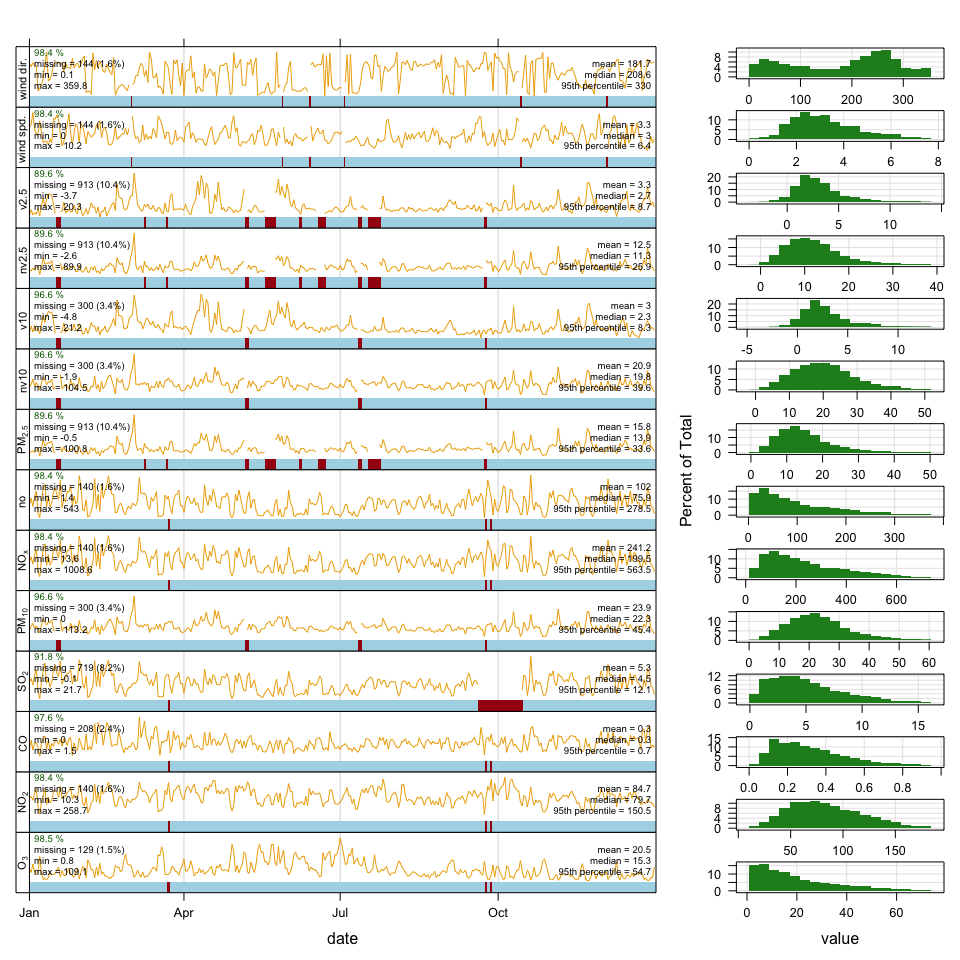# 1 Introduction

Learning R can be daunting for the novice but this does not mean that openair cannot be used in a basic way. When openair was first developed it was designed to be used by non-experts in R. In this document we will cover some basic usage to see how air quality data can be imported easily and some plots produced.

Even if you do not want to learn much R, you can still do a lot with the minimum of knowledge

Later in the course we will go through more sophisticated analyses.

# 2 Load the openair library

``library(openair)``

# 3 Import one year of data for the London Marylebone roadside site

Importing data in this way also provides hourly estimates of wind speed and wind direction from the CMAQ regional air quality model. These data should be available from part way through 2010 to the present day.

It is easy to do this in openair with one short line of code:

``my1 <- importAURN(site = "my1", year = 2018)``

# 4 Quick summary of the data

``summaryPlot(my1)``
``````##     date1     date2      code      site        o3       no2        co
## "POSIXct"  "POSIXt"  "factor"  "factor" "numeric" "numeric" "numeric"
##       so2      pm10       nox        no     pm2.5      nv10       v10
## "numeric" "numeric" "numeric" "numeric" "numeric" "numeric" "numeric"
##     nv2.5      v2.5        ws        wd
## "numeric" "numeric" "numeric" "numeric"``````# 5 Produce a time series of NO2 concentrations

``timePlot(my1, pollutant = "no2", ylab = "no2 (ug/m3)")``